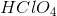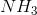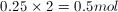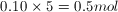GRE Subject Test: Chemistry : Indicators and Complexometry

Example Questions

Example Question #21 : Analyzing Reactions

250mL of 2Nis added to 100mL of 5N. An indicator in the solution is known to be yellow at any pH greater than 8.3 and green at any pH less than 8.3. Which of the following best describes the solution once it reaches equilibrium?

Basic and green

Neutral and green

Acidic and green

Acidic and yellow

Basic and yellow

Acidic and green

Explanation:

Each of these compounds requires one equivalent of H+ is added to 100mL of 5N NHmol, so for HClO4, 2N = 2M, and for NH3, 5N = 5M.

Using the concentrations and volumes, we can find the moles, findingHClO4 andNH3.

In this case, equivalents of acid are equal to the equivalents of base, meaning that we are at the equivalence point in a titration. HClO4 is a strong acid, and NH3 is a weak base.

Thus, the acid will fully dissociate, while the base will not, resulting in a greater concentration of H+ than OH in the solution. This means the resulting solution will be acidic. We know that the indicator changes from yellow to green at 8.3, which is a basic pH. Our initial solution is basic, and we must pass through the pH of 8.3 to reach our final acidic solution, with pH < 8.3, meaning that the indicator must change from yellow to green during the reaction. This gives out final answer that the solution will be acidic and green.

All GRE Subject Test: Chemistry Resources